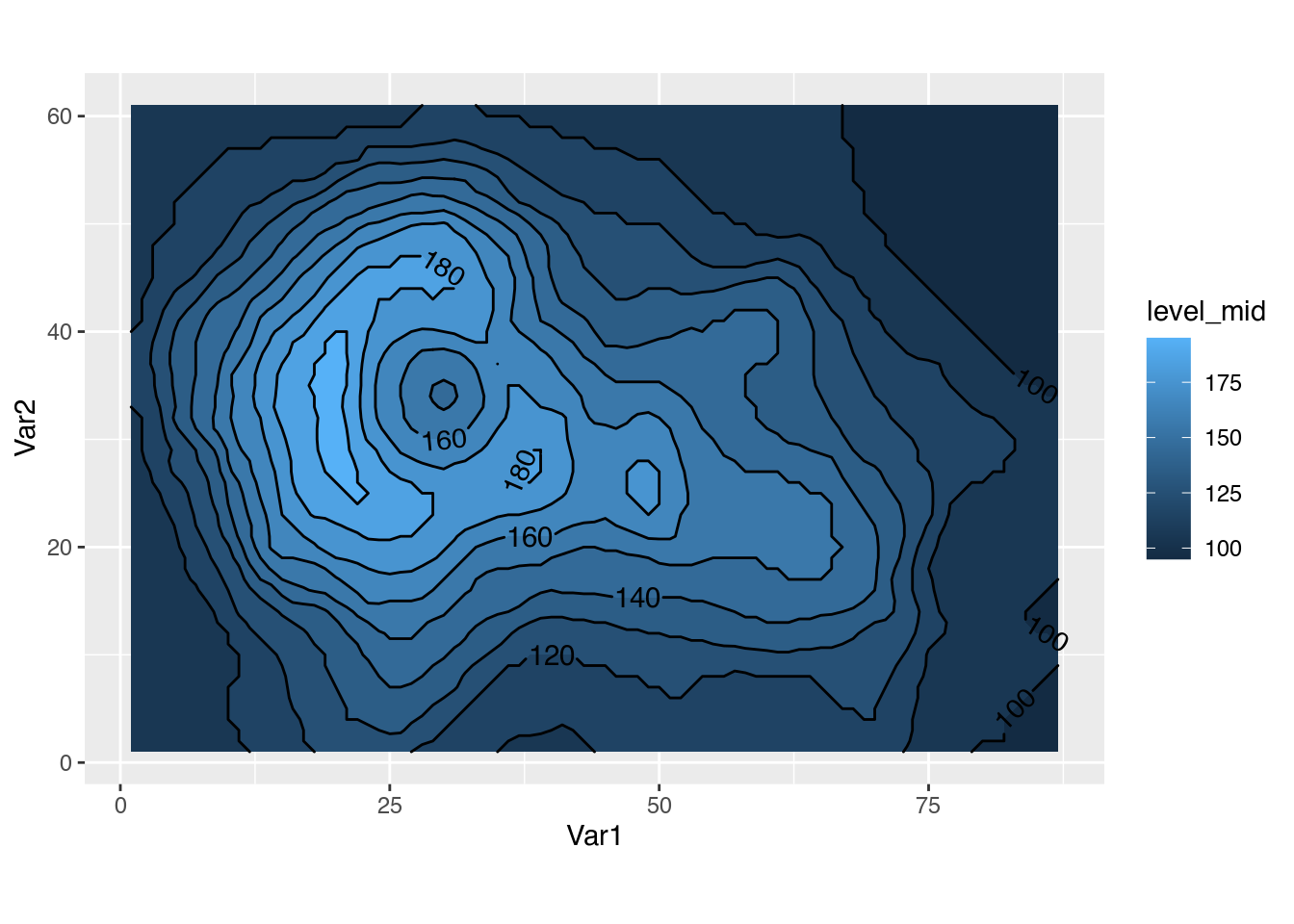I started to use R full time for my research about 5 years ago when I started working on my Masters’ thesis and up until today there was one thing missing: proper contour labels. Now, thanks to the wonderful isoband package, I finally got what I wished for and it’s bundled in the latest release of metR.

So let’s set up the stage for the problem. I have a 2D field that I want to visualise as a contour map. The canonical example in R is the volcano dataset:

library(ggplot2)
data(volcano)
volcano_df <- reshape2::melt(volcano)

With ggplot2, one would use geom_contour() like this:

ggplot(volcano_df, aes(Var1, Var2)) +
geom_contour(aes(z = value)) +
coord_equal()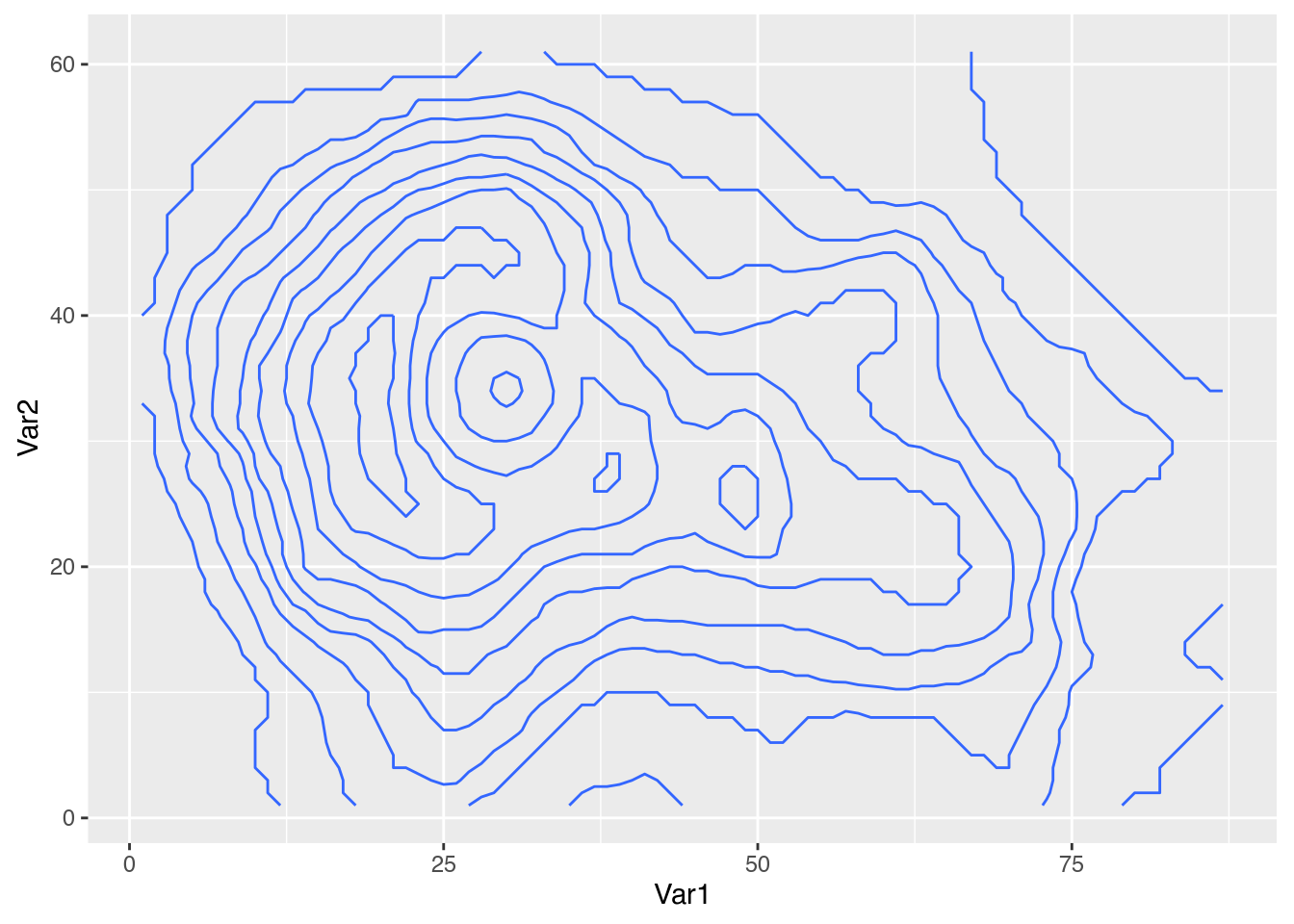With this, one can see the overall shape of the mountain but it is impossible to know the height that each contour represents. Is this an Everest-size mountain or a tiny little hill? Where is the top of the mountain? Where is the crater?

One trick is to map the colour of each line to its level like this:

ggplot(volcano_df, aes(Var1, Var2)) +
geom_contour(aes(z = value, colour = stat(level))) +
scale_colour_viridis_c() +
coord_equal()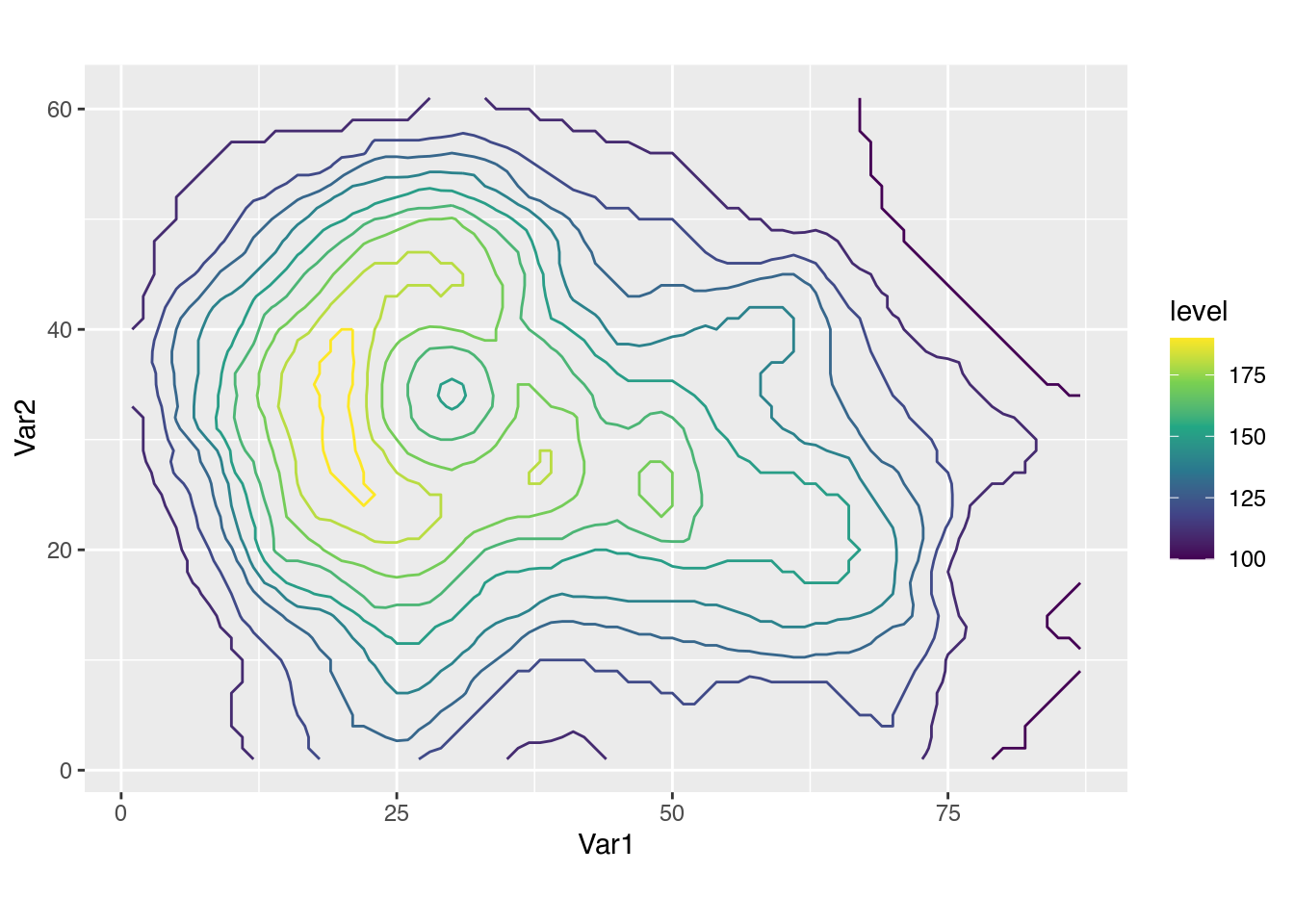This helps immensely in identifying local minimums, maximums and get a sense of the high, but it’s not super easy to pair each line with its level. There are some other tricks, but no workaround is as effective as just labelling those lines! Just look at what can be done with the contour() function.

contour(volcano)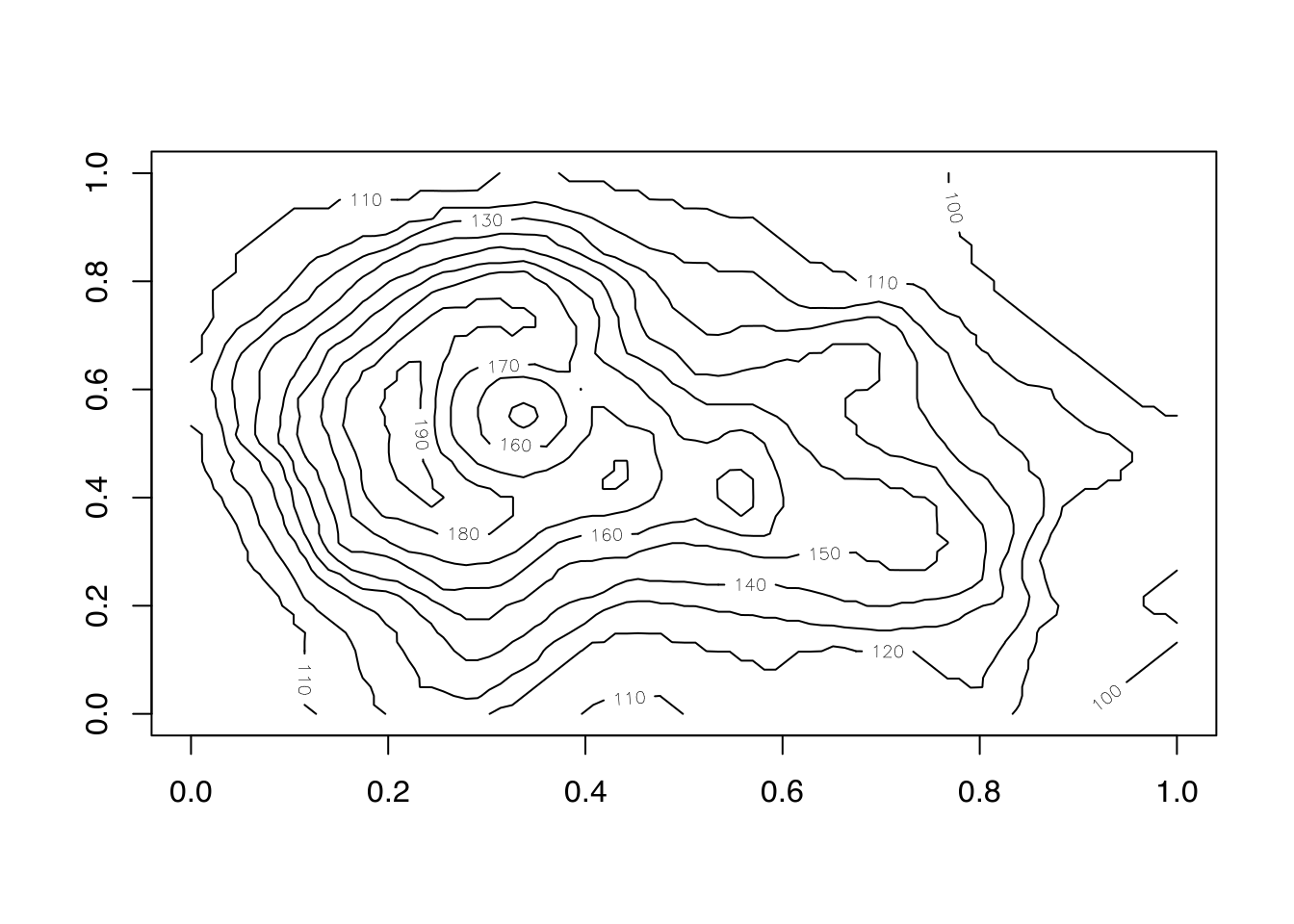With labels on each contour, it’s trivial to know the height of each point without having to mentally map colours to numbers. It would be great being able to do this with ggplot2.

One possibility is to use the old metR::geom_text_contour() function.

library(metR)      # version 0.11.0
ggplot(volcano_df, aes(Var1, Var2)) +
geom_contour(aes(z = value, colour = stat(level))) +
metR::geom_text_contour(aes(z = value)) +
coord_equal()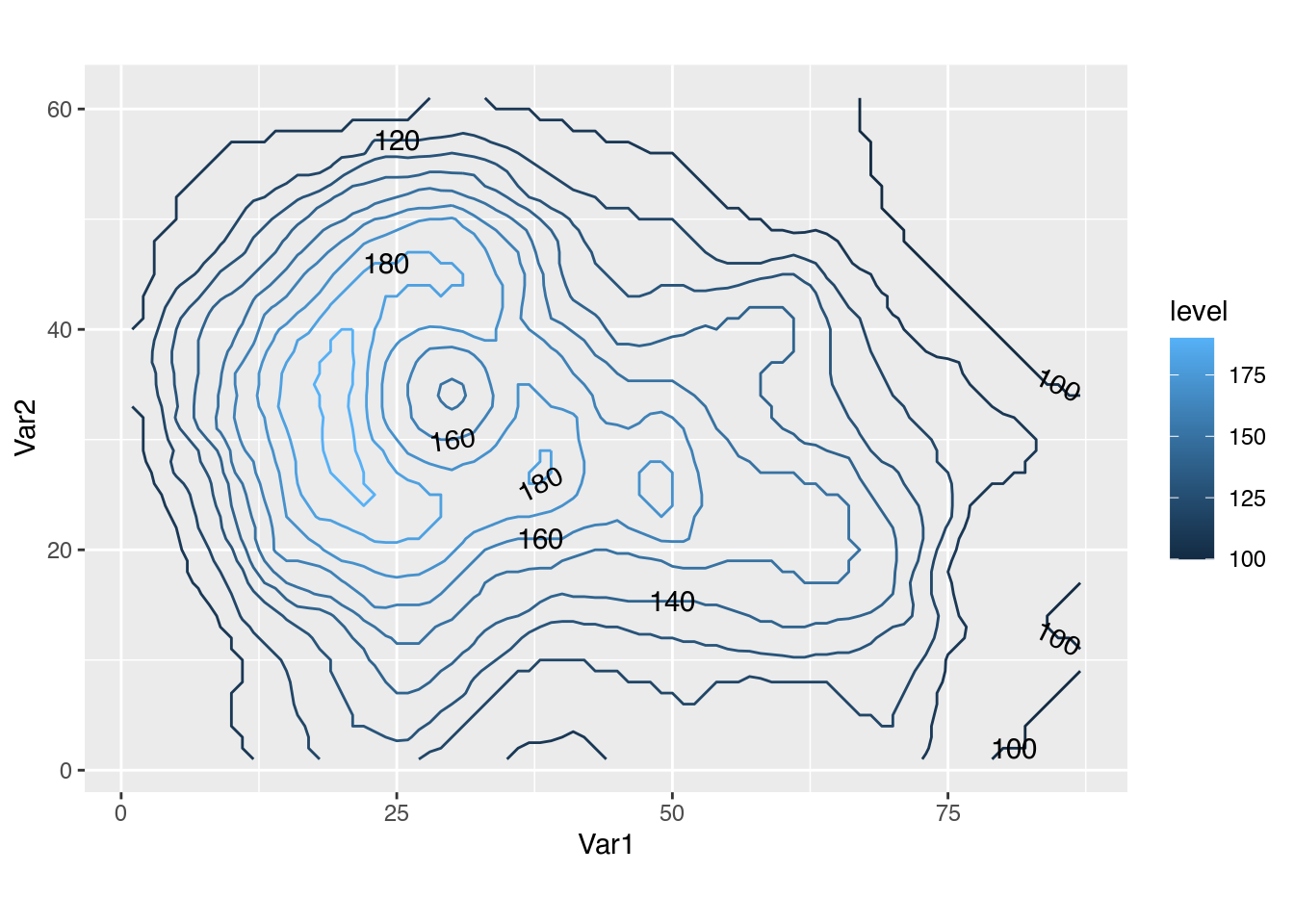By default it places a label on every second contour level looking roughly for the flattest part of the contour (label placement can be tweaked with the label.placer argument). A complicating issue is that text drawn over a line can be hard to read. One possible solution is to add a small stroke around the text so it pops against the background.

ggplot(volcano_df, aes(Var1, Var2)) +
geom_contour(aes(z = value, colour = stat(level))) +
metR::geom_text_contour(aes(z = value), stroke = 0.15) +
coord_equal()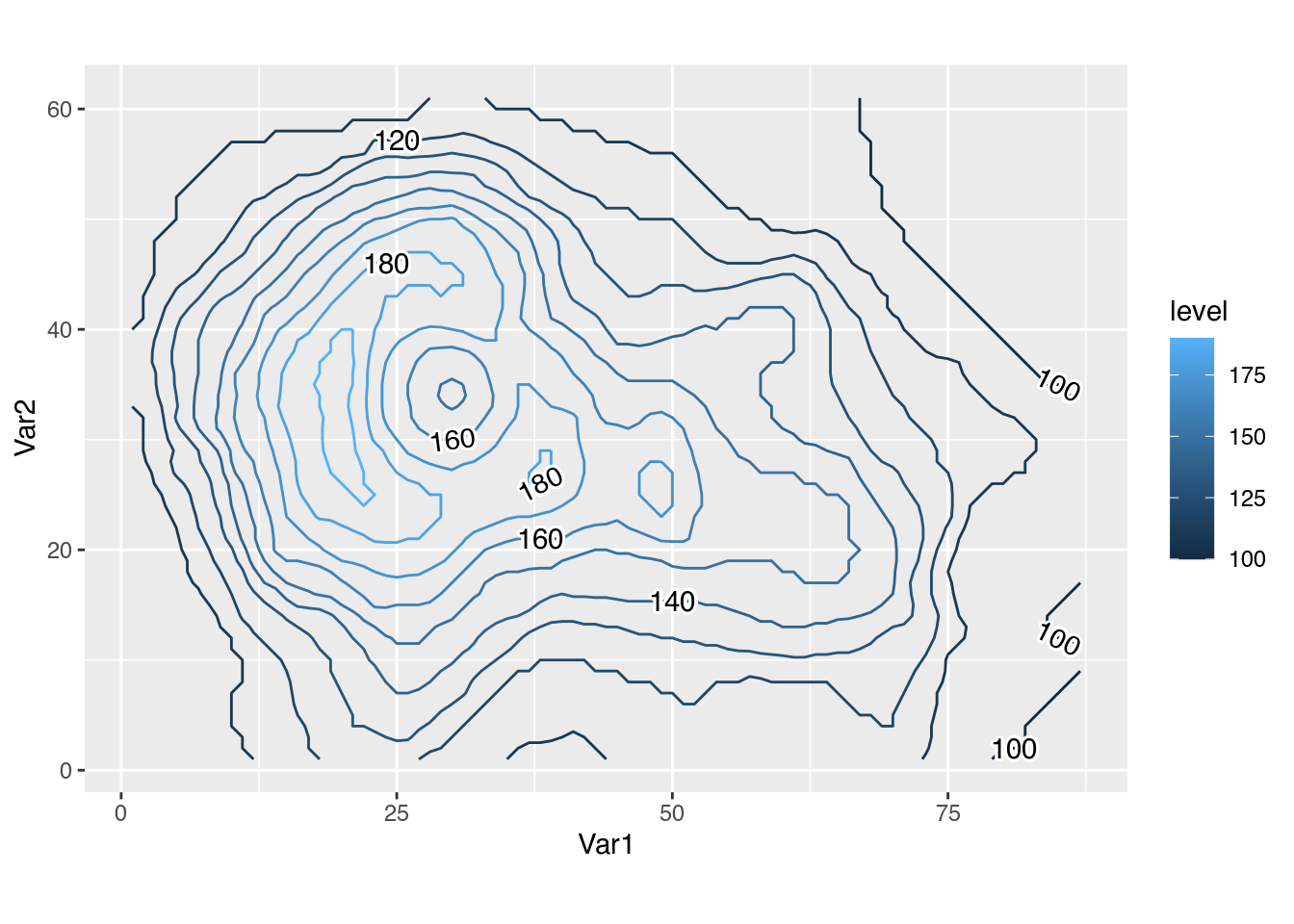But this is just a workaround and comes with its own hosts of problems. For example, it doesn’t work all that well when the background colour is not roughly uniform, such as when painting filled contours.

ggplot(volcano_df, aes(Var1, Var2)) +
metR::geom_contour_fill(aes(z = value)) +
geom_contour(aes(z = value), colour = "black") +
metR::geom_text_contour(aes(z = value), stroke = 0.15) +
coord_equal()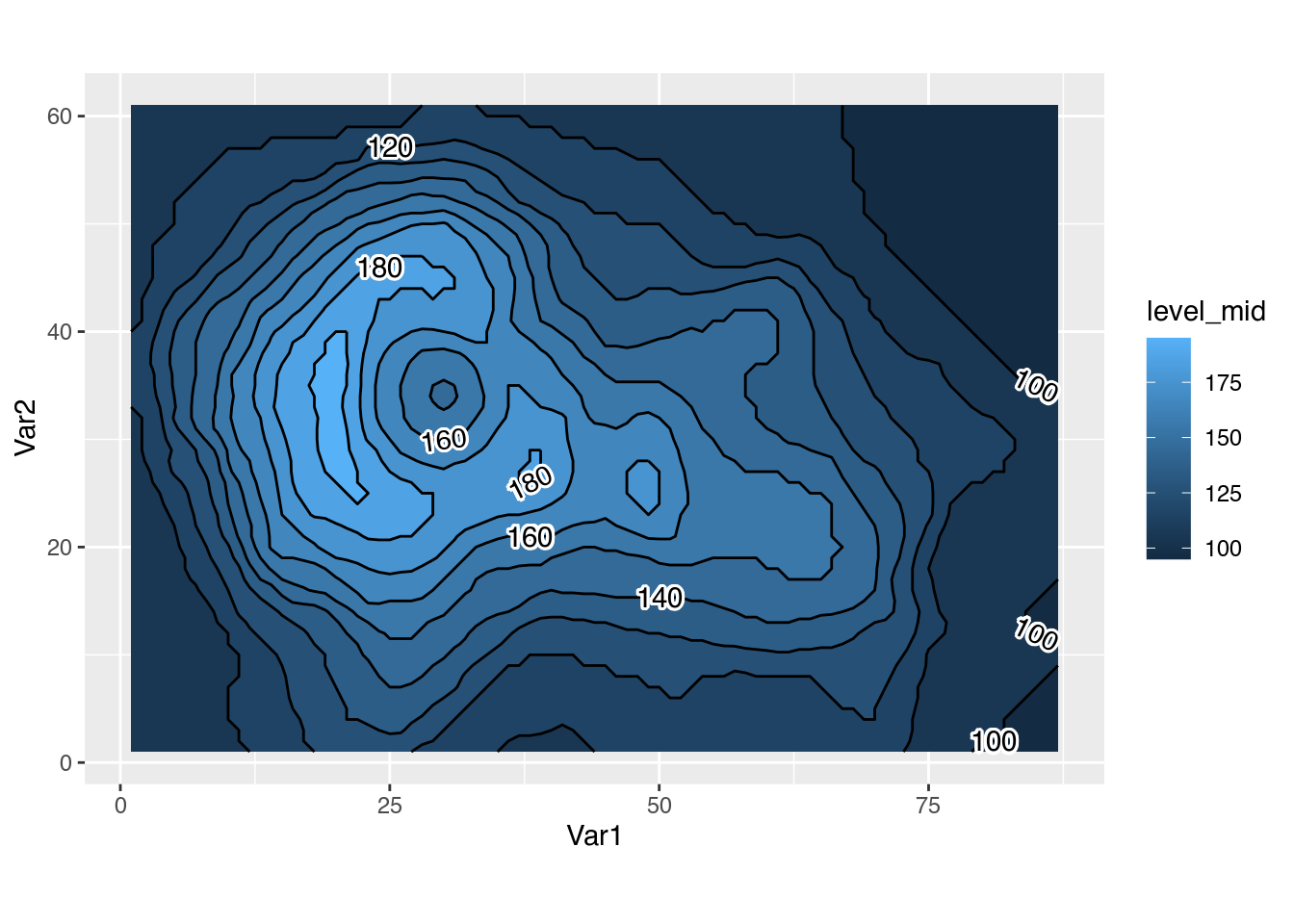The truth is that there is no substitute for actually clipping contour lines so that they don’t intersect with the text. Which is what the isoband package by Claus Wilke implements and the new version of metR bundles with its own geom_contor2(). Simply map the label aesthetic to the level computed variable and you get lovely labelled contours.

ggplot(volcano_df, aes(Var1, Var2)) +
metR::geom_contour_fill(aes(z = value)) +
metR::geom_contour2(aes(z = value, label = stat(level))) +
coord_equal()